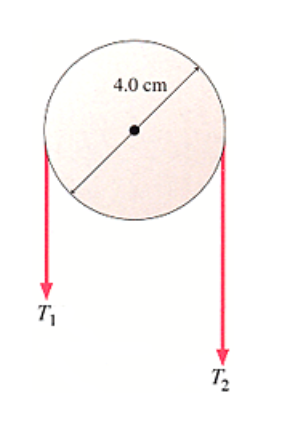# Problem: What is the net torque on the pulley about the axle if T1 = 14 N and T2 = 40 N? N m clockwise

###### FREE Expert Solution

Στ = τnet

Net torque:

τnet = rτ2 - rτ1 = r(τ2 - τ1

89% (373 ratings)###### Problem Details

What is the net torque on the pulley about the axle if T1 = 14 N and T2 = 40 N? N m clockwise